Definitions of Square Dance Calls and Concepts
Twosome Concept [C3A]

Index -->  Plus  |  A1  |  A2  |  C1  |  C2  |  C3A  |  C3B  |  C4  |  NOL  |
Definitions (Text Only) -->  Plus  |  A1  |  A2  |  C1  |  C2  |  C3A  |  C3B  |  C4  |  NOL  |
 Find call:

 \$B8@8l(BTwosome \$B\$G\$O(B, 2 \$B?M\$,\$R\$H\$^\$H\$^\$j\$GF0\$-(B (working as a unit), \$B0J2<\$N%k!<%k\$K=>\$\$\$^\$9(B:

1. \$BM?\$(\$i\$l\$?%3!<%k\$NA4BN\$rDL\$7\$F(B, Twosome \$B\$N\$^\$H\$^\$j\$N\$b\$&(B 1 \$B?M\$H(B, square \$B\$NCf\$G(B, \$BCO?^\$G\$N0LCV4X78\$rJ]\$D\$h\$&\$KF0\$-\$^\$9(B. \$B\$3\$N0UL#\$O(B, Twosome \$B\$N\$^\$H\$^\$j\$G!VKLB&!W\$G%3!<%k\$r;O\$a\$??M\$O(B, \$B%3!<%k\$NA4BN\$rDL\$7\$F!VKLB&!W\$K\$\$\$J\$1\$l\$P\$J\$i\$J\$\$\$H\$\$\$&\$3\$H\$G\$9(B.
2. Twosome \$B\$N\$^\$H\$^\$j\$N?M\$O(B 1 \$B?M\$G\$"\$k\$h\$&\$KF0\$-\$^\$9(B. \$B\$7\$?\$,\$C\$F(B, Tandem, As Couples, \$B\$^\$?\$O(B Solid \$B\$N%3%s%;%W%H\$HF1MM\$K(B, Twosome \$B\$N\$^\$H\$^\$j\$N4V\$XB>\$N?M\$rF~\$l\$?\$j(B, \$B\$^\$H\$^\$j\$r2u\$9\$3\$H\$O5v\$5\$l\$^\$;\$s(B.

\$B%3!<%i!<\$O(B, \$BIaDL(B Couples Twosome, Tandem Twosome, \$B\$^\$?\$O(B Siamese Twosome \$B\$H8@\$\$(B, \$BC/\$H0l=o\$KF0\$/\$+\$r;X<(\$7\$^\$9(B. \$B\$b\$7(B, \$BC/\$H%Z%"\$K\$J\$k\$+\$,\$"\$\$\$^\$\$\$G\$J\$1\$l\$P(B, \$B%3!<%i!<\$O(B, \$BC1\$K(B Twosome \$B\$H8@\$&\$3\$H\$b\$"\$j\$^\$9(B.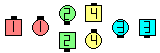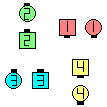(Siamese) Twosome Ah So\$B\$NA0(B \$B8e(B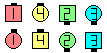\$B\$B;O\$a\$N(B formation: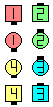Tandem TwosomeSingle Wheel\$B\$N8e(B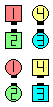Couples TwosomeSingle Wheel\$B\$N8e(B

• Twosome \$B\$K\$D\$\$\$F(B, \$B\$\$\$/\$D\$+\$N9M\$(\$,\$"\$j\$^\$9(B. \$B%"%\$%9!&%9%1!<%?!<\$N%Z%"\$NF0\$-\$r8+\$k\$H(B, 2 \$B?M\$G(B 360° \$B2s\$k\$H\$-(B, \$BKLB&\$K6a\$\$?M\$OKLB&\$K6a\$\$\$3\$H\$rJ]\$D\$h\$&\$K2s\$j(B, \$BFnB&\$K6a\$\$?M\$OFnB&\$K6a\$\$\$3\$H\$rJ]\$D\$h\$&\$K2s\$k\$3\$H\$,\$"\$j\$^\$9(B.
• Twosome \$B\$N(B 2 \$B?M\$N>e\$K2#K@\$re\$I\$&\$7\$r(B, \$BA`\$j?M7A\$N\$h\$&\$K(B, \$B;e\$G7k\$s\$G\$_\$^\$7\$g\$&(B. \$B2#K@\$O(B, \$B!V7x\$/(B -- \$B2u\$l\$J\$/(B -- \$B<'@P\$N?K\$N\$h\$&\$KF1\$8J}\$r;X\$7B3\$1\$k!W(B \$B\$H\$7\$^\$7\$g\$&(B. \$B3F!9\$N?M\$O(B, \$B<+M3\$K0l?M\$G(B, \$B<+J,\$N;e\$N2<\$G2s\$l\$^\$9\$,(B, \$B2#K@\$,;X\$7\$F\$\$\$kJ}8~\$rJQ\$(\$k\$3\$H\$O\$G\$-\$^\$;\$s(B.
• Twosome \$B\$N\$^\$H\$^\$j\$NAjo\$K<+J,\$+\$i8+\$FF1\$8J}!W\$K\$\$\$^\$9(B.
\$B%@%s%9\$N%R%s%H(B:
• Twosome \$B\$NF0\$-\$r;O\$a\$kA0\$K(B, Twosome \$B\$N\$^\$H\$^\$j\$NAj
• Twosome \$B\$rMY\$k\$H\$-\$O(B, As Couples (\$BNY\$N?M\$H\$N?M\$HJ*M}E*\$K\$D\$J\$,\$C\$F\$\$\$k\$H(B, solid \$B\$N\$^\$H\$^\$j\$G2s\$m\$&\$H\$9\$k6/\$\$798~\$,\$"\$k\$N\$G(B, \$B@5\$7\$/MY\$k\$3\$H\$,\$G\$-\$J\$/\$J\$k\$G\$7\$g\$&(B. Twosome \$B\$rMY\$k\$H\$-\$K\$O(B, \$B2s\$kF0:n\$O>o\$K(B 1 \$B?M\$G(B (individually) \$B9T\$\$\$^\$9(B.
• \$B;d\$,(B Twosome \$B\$rMY\$k\$H\$-(B, \$B\$b\$&(B 1 \$B?M\$N%@%s%5!<\$,<+J,\$K6a\$\$JI\$NJ}\$X<+J,\$r1[\$(\$F\$\$\$+\$J\$\$\$h\$&\$K(B, \$BCm0U\$r=8Cf\$5\$;\$^\$9(B. \$B;d\$N(B\$B%/%l%8%C%H%+!<%I\$r;}\$C\$F\$\$\$k:J\$r(B, \$B9b5i%G%Q!<%H\$XF~\$i\$J\$\$\$h\$&\$K\$H(B, \$B5wN%\$rCV\$\$\$F8+

\$B%3!<%k\$O(B, \$B7k6I\$N\$H\$3\$m?t>/\$J\$\$\$\$\$/\$D\$+\$N4JC1\$JF0\$-(B (\$BNc\$(\$P(B 1 \$B?M\$G1&\$^\$?\$O:8\$X(B 1/4 \$B2s\$k\$3\$H(B, Hinge \$B\$J\$I(B) \$B\$GJ,2r\$9\$k\$3\$H\$,\$G\$-\$k\$N\$G(B, \$B%@%s%5!<\$O(B, \$B0J2<\$N4pK\E*\$J(B Twosome \$B\$NF0\$-\$r\$I\$N\$h\$&\$K9T\$&\$+\$K\$D\$\$\$F(B, \$BE0DlE*\$KM}2r\$9\$Y\$-\$G\$9(B.

• Twosome Roll, 1/4 Right, 1/4 Left, \$B\$^\$?\$O(B U-Turn Back:
1 \$B?M\$GM?\$(\$i\$l\$?%3!<%k\$r\$7\$^\$9(B (\$B\$3\$l\$i\$N%3!<%k\$O\$=\$N>l\$G9T\$&\$N\$G(B).
• Tandem Twosome Hinge:
Tandem Couples \$B\$+\$i\$O(B: 1/4 In; Veer Left.
• Couples Twosome Hinge:
One-Faced Line \$B\$+\$i\$O(B: 1/2 Tag.
Two-Faced Line \$B\$+\$i\$O(B: Any Shoulder 1/2 Tag.

{n}-some (or 1 By {n}-some) [C4]: (\$B\$3\$3\$G(B n \$B\$OIaDL(B 3 \$B\$^\$?\$O(B 4): n \$B?M\$,(B, \$BB>\$HHf\$Y\$F\$*8_\$\$\$K6a\$/\$K\$\$\$F(B, \$BF1\$8J}\$r8~\$\$\$F\$\$\$F(B, \$B<+J,\$?\$A\$N4V\$KC/\$b\$\$\$J\$\$\$H\$-(B, Twosome \$B\$N%k!<%k\$K=>\$C\$F\$R\$H\$^\$H\$^\$j\$GF0\$-\$^\$9(B.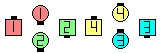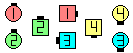1 By 3-Some Switch To A Diamond\$B\$NA0(B \$B8e(B

\$B>e\$NNc\$O Inside Triangle work 3-some: all Switch To A Diamond.

\$BCm4-some \$B\$N8@MU\$O(B, \$B\$H\$-\$K\$O(B Twosome \$B\$K4X78\$7\$J\$\$>u67\$G;H\$o\$l\$k\$3\$H\$KCm0U\$7\$F\$/\$@\$5\$\$(B. \$BNc\$(\$P(B, \$B%3!<%i!<\$,(B set \$B\$N(B each side \$B\$,0l=o\$KF0\$/\$H\$\$\$&\$3\$H\$rEA\$(\$k\$?\$a\$K(B, 'Each 4-some' \$B\$H8@\$&\$3\$H\$,\$"\$j\$^\$9(B. \$BF1MM\$NJL\$NNc\$G\$O(B, 'Interlocked Box' \$B\$N\$h\$&\$K\$b\$C\$H\$O\$C\$-\$j\$H8@\$&\$Y\$-\$H\$3\$m\$r(B, \$B\$"\$k%3!<%i!<\$O(B 'Interlocked Foursome' \$B\$N8@MU\$r;H\$\$\$^\$9(B.

Skewsome CONCEPT [C4] (Vic Ceder 1989): \$B

Dancers in a Skewsome unit: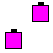or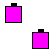Boxsome CONCEPT [C4]: 2 x 2 \$B\$K\$\$\$k%j%"%k\$N?M\$H(B phantom \$B\$,(B 4-some \$B\$GF0\$-\$^\$9(B. \$B\$3\$N%3%s%;%W%H\$O(B 2n x 2m Matrix (\$BIaDL(B 2 x 8 \$B\$^\$?\$O(B 4 x 4), \$B\$+\$i;H\$o\$l(B, \$B4pK\E*\$K\$O(B 'In Your 4 x 4 Matrix: work Boxsome...' \$B\$N\$h\$&\$K%3!<%k\$5\$l\$^\$9(B.

Skewsome | Boxsome Peel & Trail: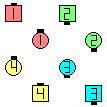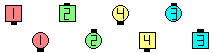Skewsome Peel & Trail\$B\$NA0(B \$B8e(B

Gruesome Twosome CONCEPT [C4]: \$BIaDL(B 2 x 8 \$B\$+\$i(B. Phantom Couples Twosome \$B\$G(B, \$BJ?9T\$J(B Wave \$B\$G\$N%3!<%k\$r9T\$&\$3\$H\$HF1\$8\$G\$9(B. Couples Twosome Phantom Waves \$B\$H\$7\$F\$bCN\$i\$l\$F\$\$\$^\$9(B.Choreography for Twosome ConceptComments? Questions? Suggestions?

https://www.ceder.net/def/twosome.php?level=C3A&language=japan
19-February-2020 00:52:07